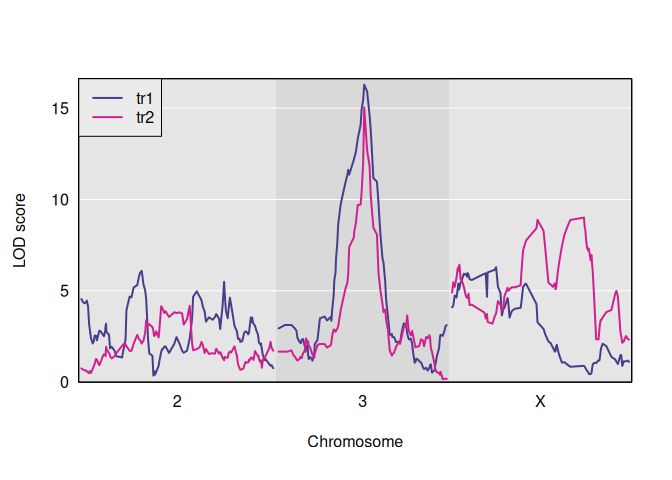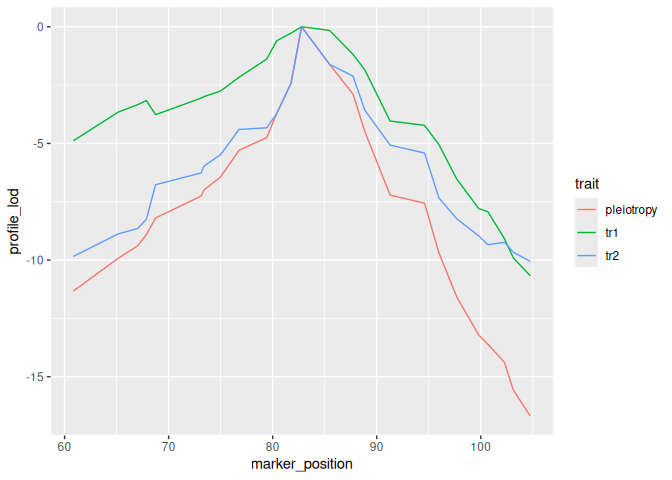# qtl2pleio

## Overview

`qtl2pleio` is a software package for use with the R statistical computing environment. `qtl2pleio` is freely available for download and use. I share it under the MIT license. The user will also want to download and install the `qtl2` R package.

Click here to explore `qtl2pleio` within a live Rstudio session in “the cloud”.

## Contributor guidelines

We eagerly welcome contributions to `qtl2pleio`. All pull requests will be considered. Features requests and bug reports may be filed as Github issues. All contributors must abide by the code of conduct.

## Technical support

For technical support, please open a Github issue. If you’re just getting started with `qtl2pleio`, please examine the vignettes on the package’s web site. You can also email frederick.boehm@gmail.com for assistance.

## Goals

The goal of `qtl2pleio` is, for a pair of traits that show evidence for a QTL in a common region, to distinguish between pleiotropy (the null hypothesis, that they are affected by a common QTL) and the alternative that they are affected by separate QTL. It extends the likelihood ratio test of Jiang and Zeng (1995) for multiparental populations, such as Diversity Outbred mice, including the use of multivariate polygenic random effects to account for population structure. `qtl2pleio` data structures are those used in the `rqtl/qtl2` package.

## Installation

To install qtl2pleio, use `install_github()` from the devtools package.

``install.packages("qtl2pleio")``

You may also wish to install the R/qtl2. We will use it below.

``install.packages("qtl2")``

## Example

Below, we walk through an example analysis with Diversity Outbred mouse data. We need a number of preliminary steps before we can perform our test of pleiotropy vs. separate QTL. Many procedures rely on the R package `qtl2`. We first load the `qtl2` and `qtl2pleio` packages.

``````library(qtl2)
library(qtl2pleio)
library(ggplot2)``````

### Reading data from `qtl2data` repository on github

We’ll consider the `DOex` data in the `qtl2data` repository. We’ll download the DOex.zip file before calculating founder allele dosages.

``````file <- paste0("https://raw.githubusercontent.com/rqtl/",
"qtl2data/master/DOex/DOex.zip")
``probs <- calc_genoprob(DOex)``

Let’s calculate the founder allele dosages from the 36-state genotype probabilities.

``pr <- genoprob_to_alleleprob(probs)``

We now have an allele probabilities object stored in `pr`.

``````names(pr)
#>  "2" "3" "X"
dim(pr\$`2`)
#>  261   8 127``````

We see that `pr` is a list of 3 three-dimensional arrays - one array for each of 3 chromosomes.

### Kinship calculations

For our statistical model, we need a kinship matrix. We get one with the `calc_kinship` function in the `rqtl/qtl2` package.

``kinship <- calc_kinship(probs = pr, type = "loco")``

### Statistical model specification

We use the multivariate linear mixed effects model:

[vec(Y) = X vec(B) + vec(G) + vec(E)]

where (Y) contains phenotypes, X contains founder allele probabilities and covariates, and B contains founder allele effects. G is the polygenic random effects, while E is the random errors. We provide more details in the vignette.

### Simulating phenotypes with `qtl2pleio::sim1`

The function to simulate phenotypes in `qtl2pleio` is `sim1`.

``````# set up the design matrix, X
pp <- pr[] #we'll work with Chr 3's genotype data``````
``````#Next, we prepare a design matrix X
X <- gemma2::stagger_mats(pp[ , , 50], pp[ , , 50])``````
``````# assemble B matrix of allele effects
B <- matrix(data = c(-1, -1, -1, -1, 1, 1, 1, 1, -1, -1, -1, -1, 1, 1, 1, 1), nrow = 8, ncol = 2, byrow = FALSE)
# set.seed to ensure reproducibility
set.seed(2018-01-30)
Sig <- calc_Sigma(Vg = diag(2), Ve = diag(2), kinship = kinship[])
# call to sim1
Ypre <- sim1(X = X, B = B, Sigma = Sig)
Y <- matrix(Ypre, nrow = 261, ncol = 2, byrow = FALSE)
rownames(Y) <- rownames(pp)
colnames(Y) <- c("tr1", "tr2")``````

Let’s perform univariate QTL mapping for each of the two traits in the Y matrix.

``s1 <- scan1(genoprobs = pr, pheno = Y, kinship = kinship)``

Here is a plot of the results.And here are the observed QTL peaks with LOD > 8.

``````find_peaks(s1, map = DOex\$pmap, threshold=8)
#>   lodindex lodcolumn chr      pos       lod
#> 1        1       tr1   3 82.77806 20.703383
#> 2        2       tr2   3 82.77806 14.417924
#> 3        2       tr2   X 48.10040  8.231551``````

### Perform two-dimensional scan as first step in pleiotropy vs. separate QTL hypothesis test

We now have the inputs that we need to do a pleiotropy vs. separate QTL test. We have the founder allele dosages for one chromosome, i.e., Chr 3, in the R object `pp`, the matrix of two trait measurements in `Y`, and a LOCO-derived kinship matrix, `kinship[]`.

``````out <- suppressMessages(scan_pvl(probs = pp,
pheno = Y,
kinship = kinship[], # 2nd entry in kinship list is Chr 3
start_snp = 38,
n_snp = 25
))``````

### Create a profile LOD plot to visualize results of two-dimensional scan

To visualize results from our two-dimensional scan, we calculate profile LOD for each trait. The code below makes use of the R package `ggplot2` to plot profile LODs over the scan region.

``````library(dplyr)
out %>%
calc_profile_lods() %>%
ggplot() + geom_line(aes(x = marker_position, y = profile_lod, colour = trait))``````### Calculate the likelihood ratio test statistic for pleiotropy v separate QTL

We use the function `calc_lrt_tib` to calculate the likelihood ratio test statistic value for the specified traits and specified genomic region.

``````(lrt <- calc_lrt_tib(out))
#>  0``````

### Bootstrap analysis to get p-values

Before we call `boot_pvl`, we need to identify the index (on the chromosome under study) of the marker that maximizes the likelihood under the pleiotropy constraint. To do this, we use the `qtl2pleio` function `find_pleio_peak_tib`.

``````(pleio_index <- find_pleio_peak_tib(out, start_snp = 38))
#> log10lik13
#>         50``````
``````set.seed(2018-11-25) # set for reproducibility purposes.
b_out <- suppressMessages(boot_pvl(probs = pp,
pheno = Y,
pleio_peak_index = pleio_index,
kinship = kinship[], # 2nd element in kinship list is Chr 3
nboot = 10,
start_snp = 38,
n_snp = 25
))``````
``````(pvalue <- mean(b_out >= lrt))
#>  1``````

## Citation information

``````citation("qtl2pleio")
#>
#> To cite qtl2pleio in publications use:
#>
#>   Boehm FJ, Chesler EJ, Yandell BS, Broman KW (2019) Testing pleiotropy
#>   vs. separate QTL in multiparental populations G3
#>   https://www.g3journal.org/content/9/7/2317
#>
#> A BibTeX entry for LaTeX users is
#>
#>   @Article{Boehm2019testing,
#>     title = {Testing pleiotropy vs. separate QTL in multiparental populations},
#>     author = {Frederick J. Boehm and Elissa J. Chesler and Brian S. Yandell and Karl W. Broman},
#>     journal = {G3},
#>     year = {2019},
#>     volume = {9},
#>     issue = {7},
#>     url = {https://www.g3journal.org/content/9/7/2317},
#>     eprint = {https://www.g3journal.org/content/ggg/9/7/2317.full.pdf},
#>   }``````